﻿ Digital Circuits and Systems - Circuits i Sistemes Digitals (CSD) - EETAC - UPCBachelor's Degree in Telecommunications Systems and in Network Engineering## Using and designing toggle flip flop (T_FF)

1. Specifications

Design a synchronous 1-bit memory cell type toggle flip-flop (T_FF). It can be used to store/save/write/register 1-bit of information, as a frequency divider by 2 or as a pulse to square wave converter. As you see, at the CLK's rising edge the input T is sampled or read, and so, if '0' the output Q maintains its previous value, and if '1' the output Q is toggled.Fig. 1. Symbol, function table and example timing diagram of a toggle  flip-flop T_FF. Clear direct (CD) is the asynchronous reset. (Visio). Another timing diagram to remember how a T_FF works.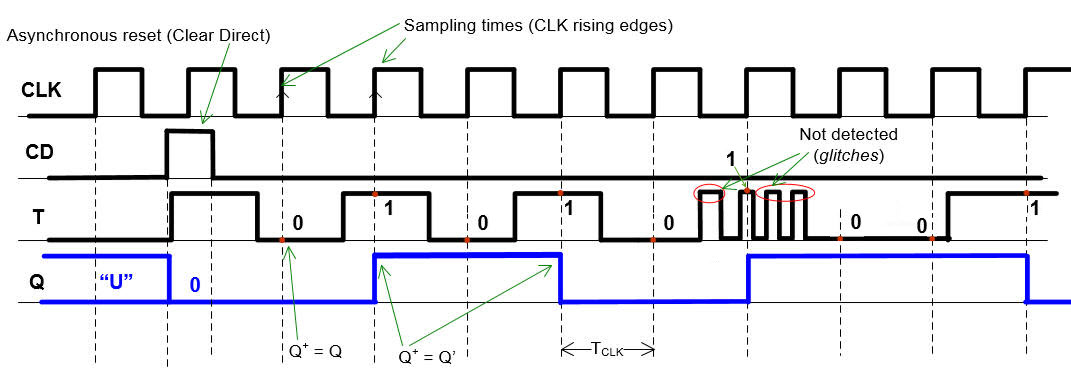2. Planning

Let's apply the FSM methodology to build and test a toggle Flip-flop (T_FF).

Thus, as you've seen in the tutorials:

1) Figure out the state diagram. Number of states and conditions of transitions. Outputs at each state.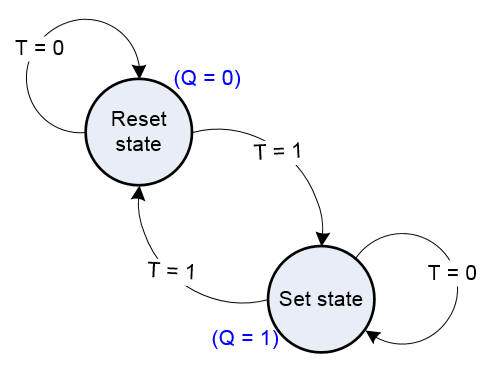Fig. 2. State diagram where the arrows indicate the transitions between states accordingly to the given condition, and in parenthesis in each state the is the indication of the output values. To make it easy, different colours are used to represent outputs and transitions.

2) Particularise the general FSM topology to this problem. The number of bits r for coding in binary the current and next states will depend on the binary code used and the number of states. [ r = number of states if one-hot code; r = (1/log 2)·log (number of states) if binary or Gray code]. In this topology you have to indicate where all the inputs and outputs are connected. In this example, because there are only 2 states, r = 1 and a single D_FF is enough for the state register.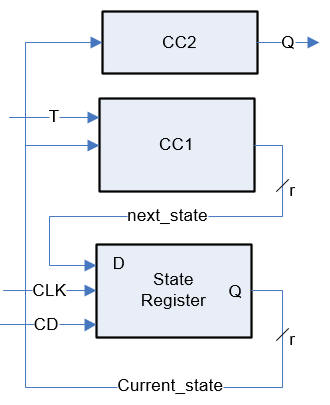Fig. 3. The topology of a finite state machine (FSM) taylored for this T_FF. We will write all the 3 components in a single file using the PROCESS statement (this is plan C1).

3) State register. We'll use always the same VHDL code description, which corresponds to a r-bit D_FF.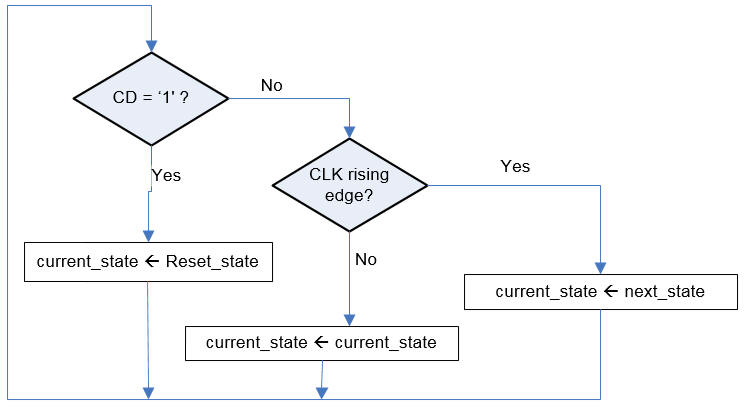Fig. 4. Flow chart for coding in VHDL the state register. It is a D_FF .

4) CC2. Truth table, and flow chart if a behavioural description is used, which is normally the best idea because Boolean algebra is left to the synthesiser. The CC2 is required to calculate the outputs at each state. The outputs in the state diagram are annotated in parenthesis and in a different colour).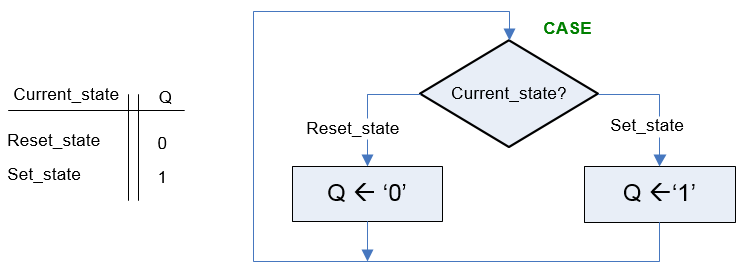Fig. 5. The truth table of the block CC2 and the flow chart of its behavioural interpretation.

5) CC1. Truth table, and flow chart if a behavioural description is used, which is normally the best idea because Boolean algebra is left to the synthesiser. CC1 is required to calculate the next state to go after the CLK's rising edge. Thus CC1 is intended to solve all the transitions (the arrows in the state diagram)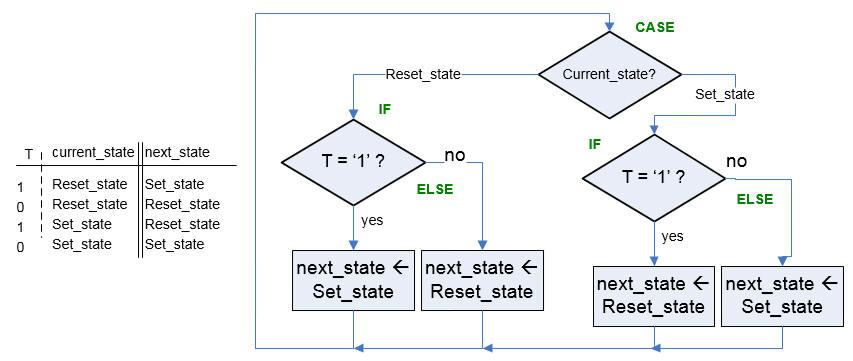Fig. 6. The truth table of the block CC1 and the flow chart of its behavioural interpretation.

6) Project folder location and file name (T_Flip_Flop.vhd):

L:\CSD\P5\T_FF/(files)

3. Development

Write down the VHDL file corresponding the the T_FF after having translated the flow charts of the CC1 and CC2 and copied the state register. Copy and adapt a previous example.

This is the T_FF.vhd.Run the EDA tool to synthesise the circuit and print and comment the RTL  schematic. Check the number of registers (DFF) used in the target chip. In this simple example, the answer is 1 register (Fig. 7). This is the circuit for the symbol in Fig. 1 with the additional output Q_L ((T_FF.vhd)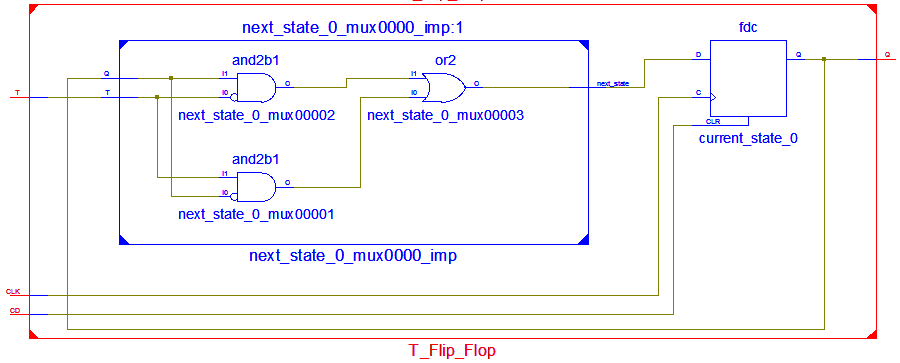Fig. 7. Example of RTL circuit implementation.

Start the test bench template and add the CLK and inputs activity translating the timing diagram. Thus, to test sequential systems at least two stimulus processes will be required: the CLK and all the other inputs. make all the timing relative to the CLK_Period constant, which is the equivalent in Chapter 2 to the Chapter 1 Min_Pulse.

4. Testing (functional, RTL view)

This in Fig. 8 is the VHDL testbench schematic that we have in mind to run simulations.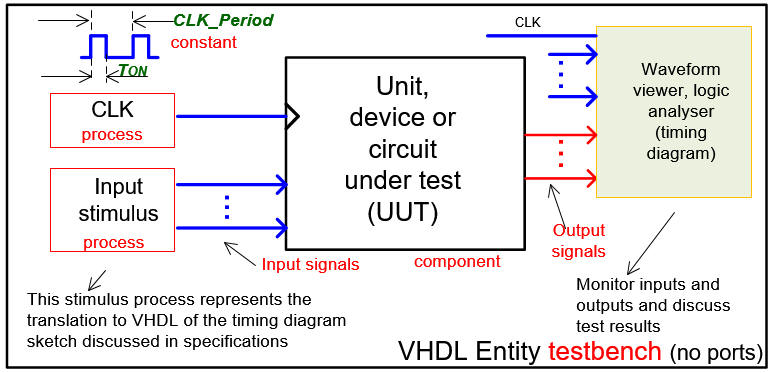Fig. 8. Testbench where to make it easy the CLK is another concurrent process. (visio)

Therefore, considering the schematic in Fig. 8, this is a sample test bench file T_FF_tb.vhd that can be used to adapt your simulations and easily modified for other synchronous applications.

Functional simulation. Run the EDA VHDL tool and demonstrate how the circuit works adding comments to the printed sheet of paper containing the waveforms.Fig. 9. Example of a  timing diagram to show how the T_FF works.

5. Testing  (gate-level and timing analyser, technology view)

Gate-level simulation. Run the EDA VHDL tool using the same test bench and demonstrate the CLK to output delays and the maximum frequency of operation.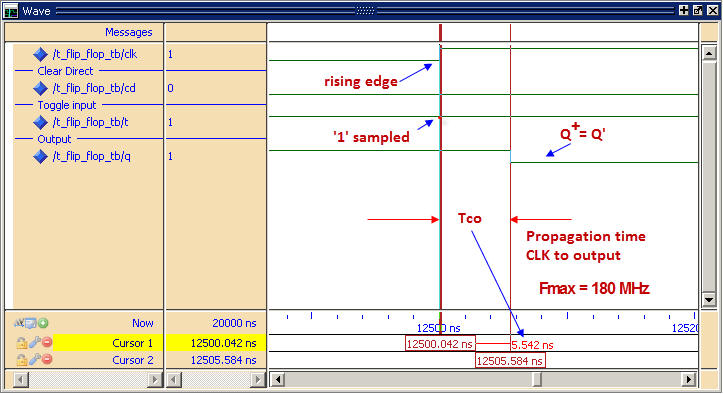Fig. 10. Example of a timing diagram to show how there is a propagation time CLK to output (Tco) which will establish the maximum frequency of operation. The target chip is an Altera MAX II EPM2210F324C3

6. Prototyping

Use training boards and perform laboratory measurements to verify how the circuit works.

7. Report

Project report starting with the template sheets of paper, scanned figures, file listings, docx , pptx, or any other resources